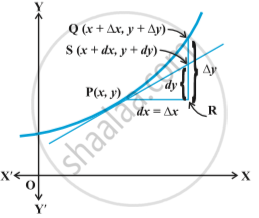# Approximations

#### notes

Let f : D → R, D ⊂ R, be a given function and let y = f(x).  Let ∆x denote a small increment in x. Recall that the increment in y corresponding to the increment in x, denoted by ∆y, is given by ∆y = f(x + ∆x) – f(x). Fig.
We define the following

(i) The differential of x, denoted by dx, is defined by dx = ∆x.

(ii) The differential of y, denoted by dy, is defined by dy = f′(x) dx or
dy =((dy)/(dx)) triangle x .

In case dx = ∆x is relatively small when compared with x, dy is a good approximation of ∆y and we denote it by dy ≈ ∆y.
For geometrical meaning of  ∆x, ∆y,  dx and  dy, one may refer to Fig.If you would like to contribute notes or other learning material, please submit them using the button below.

#### Video Tutorials

We have provided more than 1 series of video tutorials for some topics to help you get a better understanding of the topic.

Series 1

Series 2

### Shaalaa.com

Approximations and Errors [00:20:38]
S
0%